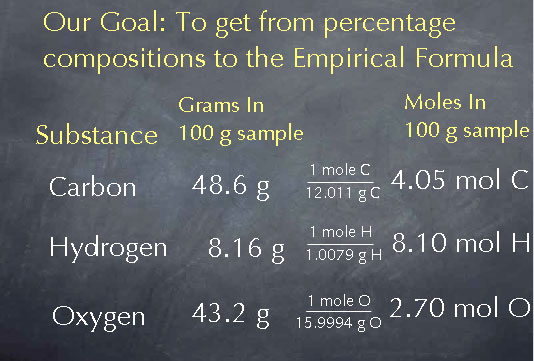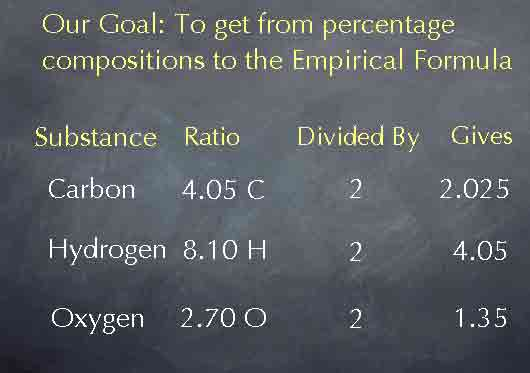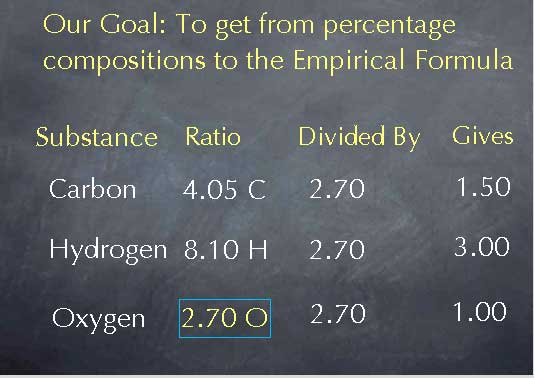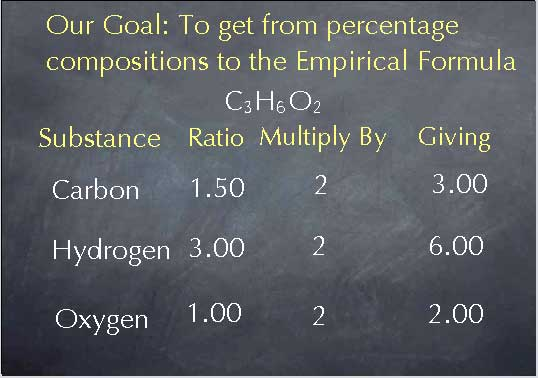# Stoichiometry Tutorials: Determining the Empirical Formula from an Elemental Analysis

(from a complete OLI stoichiometry course)

On another page, we discuss how to obtain the empirical formula from a given molecular formula. Here, we consider how to obtain the empirical formula from an elemental analysis of a sample. Remember that comparing the empirical formula obtained from an elemental analysis with that from a molecular formula tells us if the sample is consistent with the molecular formula.

### Finding the Empirical From an Elemental Analysis Movie Text

Let's say you have a sample of an unknown substance, and you want to obtain its empirical formula. You mail off the sample for analysis, and they send you a report giving the percentage of elements in the sample by mass. Our goal is to get from this to the empirical formula.

Suppose the experimental results tell us the sample is 48.6% C, 8.16% H, and 43.2% O by weight. These percentages tell us that if we had 100 grams of the sample, 48.6 grams would be carbon, 8.16 grams would be hydrogen, and 43.2 grams would be oxygen. To get the empirical formula, we need to convert from grams of carbon, hydrogen, and oxygen to the number of carbon atoms, hydrogen atoms and oxygen atoms. We can convert from grams to number of atoms (i.e. moles of atoms), by using the molecular weight. Let's first determine the number of moles of each of the elements in our 100 gram sample.

We start with the 48.6 grams of carbon per 100 grams of sample and multiply by one mole of carbon per every 12.011 grams of carbon to get 4.05 moles of carbon per 100 grams of sample. Similarly for hydrogen, we start with 8.16 grams of hydrogen, multiply by one mole of hydrogen per every 1.0079 grams of hydrogen, and get 8.10 moles of hydrogen in 100 grams of sample. Finally, for oxygen, we find that (43.2 grams O) (1 mol O/ 15.9994 g O) gives us 2.70 mol O per 100 g sample.We've now obtained the number of atoms of C, H and O in 100 g of the sample. This gets us partway to our goal of calculating the empirical formula. However, the empirical formula is related to the ratios between the quantity of these elements, so we will next calculate these ratios. The ratio of C atoms: H atoms: O atoms is 4.05 : 8.10 : 2.70.To obtain the empirical formula, we need to reduce these ratios to their lowest whole numbers. To do this, we'll take advantage of the fact that we can multiply or divide these numbers by anything we like while keeping the ratio the same. For instance, if we divide by two, cutting all of the numbers in half, we get the results you see on the screen below, and we haven't changed the ratios between the number of atoms.Dividing by 2 wasn't particularly useful, but suppose we divide each of these numbers by 2.70, the smallest of the three numbers. Then we get the results you see below. The ratio now becomes 1.50 to 3.00 to 1.00. By choosing the smallest of our original three numbers, we have made one of the numbers in the ratio a 1 and simplified the other numbers. We are now very close to having the empirical formula; we know that there are 1.50 carbon atoms for every 3.00 hydrogen atoms and 1.00 oxygen atom.The empirical formula should present the ratios between elements in terms of the lowest whole numbers. To get to the lowest whole number ratio, we can multiply each of the numbers by 2 to get 3.00 : 6.00: 2.00. Multiplying by 2 converted 1.50 into the whole number 3.00. This now tells us the ratio between the atoms of each element in terms of the smallest whole number ratios, leading to the empirical formula C3H6O2.You may now try a tutor on deriving the empirical formula from an elemental analysis of a mineral ore.